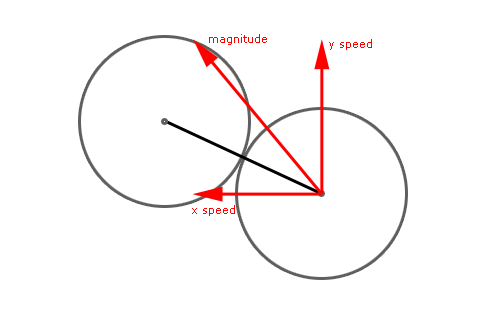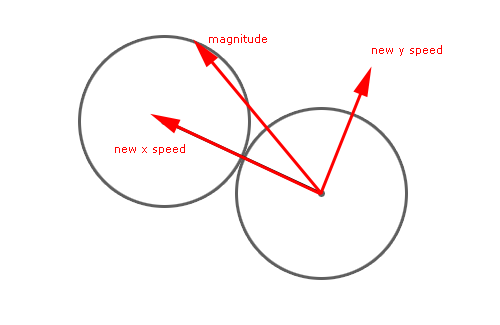# Managing ball vs ball collision with Flash

This is another headache post. I am sorry, but since there is a ball physics competition, I have to focus tutorials on ball physics.

This tutorial covers collisions between balls, and it works with any number of balls, and it’s based on Raman Pfaff’s Physics of an Elastic Collision.

What is an “elastic collision”?

From Wikipedia: “An elastic collision is a collision in which the total kinetic energy of the colliding bodies after collision is equal to their total kinetic energy before collision.”Then, in the first frame, just eighty lines of actionscript:

```number_of_balls = 3;
speed_limit = 10;
for (x=1; x<=number_of_balls; x++) {
ball = attachMovie("ball", "ball_"+x, _root.getNextHighestDepth(), {_x:Math.random()*400-50, _y:Math.random()*300-50});
ball.collision = 0;
ball.mass = 1;
ball.xspeed = Math.random()*8-4;
ball.yspeed = Math.random()*8-4;
ball.onEnterFrame = function() {
if (this._x<15) {
this._x = 15;
this.xspeed *= -1;
}
else if (this._x>485) {
this._x = 485;
this.xspeed *= -1;
}
if (this._y<15) {
this._y = 15;
this.yspeed *= -1;
}
else if (this._y>385) {
this._y = 385;
this.yspeed *= -1;
}
if (this.xspeed>speed_limit) {
this.xspeed = speed_limit;
}
if (this.xspeedspeed_limit) {
this.yspeed = speed_limit;
}
if (this.yspeed30) {
_root["ball_"+x].collision = 0;
_root["ball_"+y].collision = 0;
}
}
}

};```

Let’ see how does it work

Line 1: Defining the number of balls in the stage. Placing an high number of balls can cause some problems, as I will explain later

Line 2: Setting a speed limit. It’s not necessary in this example but we should always set a speed limit. The higher the speed, the lower the accuracy of our collisions.

Line 3: Beginning of a cycle to be repeated n times, where n = number of balls in the stage

Line 4: Attaching the ball on the stage in a random position. Please note that when I place a ball, I don’t check if it will overlay a previously placed ball. This is why placing an high number of balls can cause some problems, but placing “safe” balls is not the aim of this tutorial. Moreover, you can easily script the routine to check if two balls overlay.

Line 5: Setting a collision property to the ball. Collision = 0 means there is no collisions

Line 6: Mass property. Having all same masses helps us to verify the script works… but you can set the mass at any value, and the script will work. It’s just that you’ll see some strange bounces and you won’t understand why since the balls in the stage do not display their mass.

Lines 7-8: Assigning a random x and y speeds to the ball

Line 9: Beginning of the routine to be executed at every frame for each ball

Lines 10-13: Checking if the ball is closer than 15 pixels (its radius) to the left edge of the stage. If so, it means the ball must “bounce” on the edge, so its x position is set to 15 and its x speed is inverted.

Lines 14-17: Same thing with the right edge

Lines 18-21: Same thing with the upper edge

Lines 22-25: Same thing with the bottom

Lines 26-28: Checking if the ball xspeed exceeds speed limits. If true, setting xspeed to speed limit

Lines 29-31: Same thing with negative x speed (from right to left)

Lines 32-37: Same thing with y positive and negative speeds

Lines 38-39: Updating ball position according to x and y speeds

Well, I told the script was 80 lines long (81 to be precise)… we are at its half and we did not encounter hard points. Unfortunately, this will not last for long…

Lines 42-62: Mysterious routine that solves the collision between two balls updating their speeds according to various collision parameters. At the moment you don’t need to know more.

Line 63: Function to be executed at every frame

Lines 64-65: This is a double cycle used to check the collision between a ball and the other ones. If you look at the parameters of both cycles, you’ll notice they are designed to avoid checking collision twice. For example, I will never check collision between ball 2 and ball 1, because I already checked collision between ball 1 and 2. So, if we have 4 balls, the collision checks will be: ball 1 with 2, 1 with 3, 1 with 4, 2 with 3, 2 with 4 and 3 with 4.

Lines 66-68: Determining distance between xth and yth balls with the Pytagorean theorem

Line 69: Checking if the distance is less than 30 pixels (ball diameter, or 2*ball radius) and both balls are not already colliding.

Why are we checking if balls are already in collision? Because we want an elastic collision, and an elastic collision must occour only at a distance of 30 pixels. Unfortunately, we can’t check the distance between balls in a continuous time, but only every 1/framerate seconds, so in most cases we miss the moment when two balls start colliding… in most cases the collision begins when a ball overlayed another one and we have to avoid “fake bounces” as the ones explained in Create a flash draw game like Line Rider or others – part 4… so the entire process is an approximation of a real elastic collision, but this won’t affect gameplay.

Lines 70-71: Set the collision propery of both balls to 1

Line 72: Calling the “mysterious routine”

Lines 74-77: If the distance is more than 30px, then there is no collision and I set the collision property to zero. This is not necessary in most cases but I leave to you the code optimization.

That’s all! Did I forget something? What? The Mysterious routine?

Line 42: Function declaration: two arguments, both movieclips, both balls

Line 43: Calculating the horizontal distance between balls

Line 44: Calculating the vertical between balls

Line 45: Calculating the collision angle using trigonometry as explained in Create a flash draw game like Line Rider or others – part 3

Line 46: Calculating the ball1 speed, here called “magnitude” because this is the “real name” of the speed in this environment

Line 47: Calculating the ball2 speed in the same way

Lines 48-49: Determining balls direction using trigonometry

Nothing new until here, if you are a regular reader. Now, let’s mess up everything.

That’s what we have: a collision of 2 balls in a standard reference axis system, with an horizontal and a vertical speed that form the magnitude like in this pictureAs you can see, y speed is vertical while x speed is horizontal.

Now imagine to change reference axis system, where the x speed lies along the collision line, and the y speed is perpendicular to that, without changing magnitude and direction, in this wayBoth pictures show the concept on the right ball, but it’s the same for both balls

All variables we need to know are known, so new speeds are determined this way:

Line 50: Calculating new xspeed using trigonometry applied to the difference from the direction angle and the collision angle (in other words, how many degrees it takes to make the xspeed vector overlay the angle of collision)

Lines 51-54: Same thing for other vectors: ball 1 yspeed and ball 2 xspeed and yspeed

Now new vectors are known. It’s time to calculate what happens in this collision. Let’s think about the collision we have: balls are moving on a 2D environment, but with this axis transformation the collision happens in a 1D environment: along the x axis.

The only thing we need to solve is an elastic collision along one axis.

Just like Superman, the law of conservation of momentum comes in our help (and without blue/red underpants).

From Wikipedia: In an isolated system (one where external forces are absent) the total momentum will be constant: this is implied by Newton’s first law of motion. Newton’s third law of motion, the law of reciprocal actions, which dictates that the forces acting between systems are equal in magnitude, but opposite in sign, is due to the conservation of momentum.

Do we have an isolated system? Yes, external forces are absent, it’s windy today in Italy but the wind does not affect my spheres in the stage.

So our friend Newton says in an elastic collision total kinetic energy is the same before and after the collision and total momentum remains constant throughout the collision. Calling v1 and v2 the new magnitudes (speeds) of the balls after the collision, u1 and u2 the magnitudes before collision, and m1 and m2 the masses, we have:

v1 = (u1*(m1-m2) + 2*m2*u2)/(m1+m2)

and

v2 = (u2*(m2-m1) + 2*m1*u1)/(m1+m2)

Notice that if m1=m2 then

v1 = u2

and

v2 = u1

That means the balls exchange their magnitude.

Translated into actionscript, this is:

Line 54: Determining final x speed for ball 1

Line 55: Determining final y speed for ball 2

Lines 56-57: y speed does not changes (it’s a 1D collision)

And now we have the new x and y speeds, but they are still related to the axis environment we created to have a 1D collision.

Time to transform speeds in the old, standard x-y Cartesian coordinate system

Lines 58-61: Determining x and y speeds on the standard axis system using trigonometry. Notice that `Math.PI/2` because the angle between xspeed and yspeed must always be 90 degrees (pi/2 radians)

And the problem is solved… here you can see three balls with equal masses bouncing up and down the stage. Should you see two balls overlaying, that’s due to the wrong initial placing as explaining at the beginning of this tutorial. Simply reload the page and everything should work.

214 GAME PROTOTYPES EXPLAINED WITH SOURCE CODE
// 1+2=3
// 10000000
// 2 Cars
// 2048
// Avoider
// Ballz
// Block it
// Blockage
// Bloons
// Boids
// Bombuzal
// Breakout
// Bricks
// Columns
// CubesOut
// Dots
// DROP'd
// Dudeski
// Eskiv
// Filler
// Fling
// Globe
// HookPod
// Hundreds
// InkTd
// Iromeku
// Lumines
// Magick
// MagOrMin
// Maze
// Memdot
// Nano War
// Nodes
// o:anquan
// Ononmin
// Pacco
// Phyballs
// Platform
// Poker
// Pool
// Poux
// Pudi
// qomp
// Racing
// Renju
// SameGame
// Security
// Sling
// Slingy
// Sokoban
// Splitter
// Sproing
// Stack
// Stringy
// Sudoku
// Tetris
// Threes
// Toony
// Turn
// TwinSpin
// vvvvvv
// Wordle
// Worms
// Yanga
// Zhed
// zNumbers